# Finance Bill (HL Bill 71)

Finance BillPage 320

• B is the aggregate of the interest allowances of the group for
periods before the current period so far as they are available
in the current period (see section 393).

(2) Where the amount determined under subsection (1) is less than the
5de minimis amount for the current period, the interest capacity of the
worldwide group for the period is the de minimis amount.

(3) For this purpose “the de minimis amount” for a period of account
is—

(a) £2 million, or

(b) 10where the period is more than or less than a year, the amount
mentioned in paragraph (a) proportionately increased or
reduced.

###### 393Amount of interest allowance for a period that is “available” in a later period

(1) 15This section applies for the purposes of this Chapter.

(2) The amount of the interest allowance of a worldwide group for a
period of account (“the originating period”) that is “available” in a
later period of account of the group (“the receiving period”) is
(subject to subsection (5)) the lower of amounts A and B.

(3) 20Amount A is—

(a) the amount of the interest allowance for the originating
period, less

(b) the total of the amount or amounts (if any) of that interest
allowance that were used in the originating period, or in any
25subsequent period of account of the group before the
receiving period (see section 394).

(4) Amount B is the amount (if any) of the interest allowance for the
originating period that is unexpired in the receiving period (see
section 395).

(5) 30The amount of the interest allowance for the originating period that
is “available” in the receiving period is nil if—

(a) an abbreviated return election is made in relation to the
originating period, the receiving period or any intervening
period of account of the group, or

(b) 35an interest restriction return is not submitted for any such
period.

###### 394When interest allowance is “used”

(1) This section applies for the purposes of this Chapter.

(2) The amount of the interest allowance of a worldwide group for a
40period of account of the group (“the originating period”) that is
“used” in the originating period is the lower of—

(a) the interest allowance for the originating period, and

(b) the sum of—

(i) the aggregate net tax-interest expense of the group for
45the originating period;

Finance BillPage 321

(ii) the total amount of tax-interest expense amounts
required to be brought into account in the originating
period under section 379 (reactivation of interest) by
members of the group.

(3) 5The amount of the interest allowance for the originating period that
is “used” in a later period of account of the group (“the receiving
period”) is the lower of—

(a) the interest allowance so far as it is available in the receiving
period (see section 393), and

(b) 10the relevant part of the aggregate net tax-interest expense of
the group for the receiving period (see subsection (4)).

(4) In subsection (3)(b) “the relevant part of the aggregate net tax-
interest expense of the group for the receiving period” is (subject to
subsection (5))—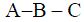15

where—

A is the aggregate net tax-interest expense of the group for the
receiving period;

B is the interest allowance of the group for the receiving period;

20C is the amount of the interest allowance of the group for any
period before the originating period that is used in the
receiving period.

(5) Where the amount determined under subsection (4) is negative, “the
relevant part of the aggregate net tax-interest expense of the group
25for the receiving period” is nil.

###### 395Amount of interest allowance for a period of account that is “unexpired” in later period

(1) This section contains provision for determining for the purposes of
this Chapter the extent to which an interest allowance of a
30worldwide group for a period of account (“the originating period”)
is “unexpired” in a later period of account of the group (“the
receiving period”).

(2) If the receiving period—

(a) begins 5 years or less after the originating period begins, and

(b) 35ends 5 years or less after the originating period ends,

all of the interest allowance for the originating period is unexpired in
the receiving period.

(3) If the receiving period begins 5 years or more after the originating
period ends, none of the interest allowance for the originating period
40is unexpired in the receiving period.

(4) Subsection (5) applies if the receiving period—

(a) begins more than 5 years after the originating period begins,
and

(b) ends 5 years or less after the originating period ends.

Finance BillPage 322

(5) The amount of the interest allowance for the originating period that
is unexpired in the receiving period is—where—

5A is the interest allowance for the originating period;

B is—

(a)

the aggregate net tax-interest expense of the group for
the originating period, or

(b)

if lower, the interest allowance for the originating
10period;

X is the number of days in the period—

(a)

beginning with the day on which the receiving period
begins, and

(b)

ending with the day 5 years after the day on which the
15originating period ends;

Y is the number of days in the originating period.

(6) Subsection (7) applies if the receiving period—

(a) begins 5 years or less after the originating period begins, and

(b) ends more than 5 years after the originating period ends.

(7) 20The amount of the interest allowance for the originating period that
is unexpired in the receiving period is—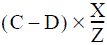where—

C is the aggregate net tax-interest expense of the group for the
25receiving period;

D is—

(a)

the interest allowance of the group for the receiving
period, or

(b)

if lower, the aggregate net tax-interest expense of the
30group for the receiving period;

X has the same meaning as in subsection (5);

Z is the number of days in the receiving period.

(8) Subsection (9) applies if—

(a) the receiving period—

(i) 35begins more than 5 years after the originating period
begins, and

(ii) ends more than 5 years after the originating period
ends, and

(b) subsection (3) does not apply.

(9) 40The amount of the interest allowance for the originating period that
is unexpired in the receiving period is the lower of the amounts
determined under subsections (5) and (7).

Finance BillPage 323

##### CHAPTER 5Interest allowance
###### 396The interest allowance of a worldwide group for a period of account

(1) 5For the purposes of this Part “the interest allowance” of a worldwide
group for a period of account of the group is—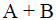where—

A is the basic interest allowance of the group for the period;

10B is the amount (if any) of the aggregate net tax-interest income
of the group for the period (see section 390(3) and (4)).

(2) In subsection (1) “the basic interest allowance” means—

(a) where no group ratio election is in force in relation to the
period, the basic interest allowance calculated using the fixed
15ratio method (see section 397);

(b) where such an election is in force in relation to the period, the
basic interest allowance calculated using the group ratio
method (see section 398).

###### 397Basic interest allowance calculated using fixed ratio method

(1) 20For the purposes of section 396, the basic interest allowance of a
worldwide group for a period of account of the group, calculated
using the fixed ratio method, is the lower of the following amounts—

(a) 30% of the aggregate tax-EBITDA of the group for the period;

(b) the fixed ratio debt cap of the group for the period.

(2) 25See—

• section 400 for the meaning of “fixed ratio debt cap”;

• section 405 for the meaning of “aggregate tax-EBITDA”.

###### 398Basic interest allowance calculated using group ratio method

(1) For the purposes of section 396, the basic interest allowance of a
30worldwide group for a period of account of the group, calculated
using the group ratio method, is the lower of the following
amounts—

(a) the group ratio percentage of the aggregate tax-EBITDA of
the group for the period;

(b) 35the group ratio debt cap of the group for the period.

(2) See—

• section 399 for the meaning of “group ratio percentage”;

• section 400 for the meaning of “group ratio debt cap”;

• section 405 for the meaning of “aggregate tax-EBITDA”.

Finance BillPage 324

###### 399The group ratio percentage

(1) For the purposes of this Part “the group ratio percentage” of a
worldwide group for a period of account of the group is (subject to
subsection (2)) the following proportion expressed as a percentage—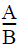5

where—

A is the qualifying net group-interest expense of the group for
the period;

B is the group-EBITDA of the group for the period.

(2) 10“The group ratio percentage” is 100% where—

(a) the percentage determined under subsection (1) is negative
or higher than 100%, or

(b) B in that subsection is zero.

(3) See—

• 15section 414 for the meaning of “qualifying net group-interest
expense”;

• section 416 for the meaning of “group-EBITDA”.

###### 400The debt cap

(1) For the purposes of section 397 (and this section), “the fixed ratio
20debt cap” of a worldwide group for a period of account of the group
is the sum of the following amounts—

(a) the adjusted net group-interest expense of the group for the
period;

(b) the excess debt cap of the group that was generated in the
25immediately preceding period of account of the group (if
any) (see subsections (3) to (7)).

(2) For the purposes of section 398 (and this section), “the group ratio
debt cap” of a worldwide group for a period of account of the group
is the sum of the following amounts—

(a) 30the qualifying net group-interest expense of the group for the
period;

(b) the excess debt cap of the group that was generated in the
immediately preceding period of account of the group (if
any) (see subsections (3) to (7)).

(3) 35Where no group ratio election is in force in relation to a period of
account of a worldwide group (“the generating period”), “the excess
debt cap” of the group that is generated in the period is (subject to
subsections (5) and (6))—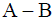40where—

A is the fixed ratio debt cap of the group for the generating
period;

B is 30% of the aggregate tax-EBITDA of the group for the
generating period.

Finance BillPage 325

(4) Where a group ratio election is in force in relation to a period of
account of a worldwide group (“the generating period”), “the excess
debt cap” of the group that is generated in the period is (subject to
subsections (5) and (6))—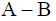5

where—

A is the group ratio debt cap of the group for the generating
period;

B is the group ratio percentage of the aggregate tax-EBITDA of
10the group for the generating period.

(5) Where the amount determined under subsection (3) or (4) is
negative, “the excess debt cap” of the group that is generated in the
period is nil.

(6) Where the amount determined under subsection (3) or (4) is greater
15than the carry-forward limit, “the excess debt cap” of the group that
is generated in the period is the carry-forward limit.

(7) For this purpose the “carry-forward limit” is the sum of the following
amounts—

(a) the excess debt cap generated in the period of account of the
20group immediately preceding the generating period (if any);

(b) the total disallowed amount of the group in the generating
period.

(8) See—

• section 373 for the meaning of “the total disallowed amount”;

• 25section 405 for the meaning of “aggregate tax-EBITDA”;

• section 413 for the meaning of “adjusted net group-interest
expense”;

• section 414 for the meaning of “qualifying net group-interest
expense”.

###### 401Effect of group ratio (blended) election on group ratio percentage

(1) Where a group ratio (blended) election (see paragraph 14 of Schedule
7A) has effect in relation to a period of account of a worldwide group
(“the relevant period of account”), this Chapter applies subject to this
35section.

(2) Section 399 (meaning of “group ratio percentage”) does not apply for
the purpose of determining the group ratio percentage of the group
for the relevant period of account.

(3) Instead, the group ratio percentage of the group for the relevant
40period of account is determined by taking the following steps—

Step 1

For each investor in the group, multiply the investor’s applicable
percentage by the investor’s share in the group.

Step 2

45Add together the amounts found under Step 1.

Finance BillPage 326

(4) For the purposes of this section, an investor’s “applicable
percentage” is the highest of the following percentages—

(a) 30%;

(b) the percentage determined under section 399;

(c) 5in the case of a related party investor that, throughout the
relevant period of account, is a member of a worldwide
group (“the investor’s worldwide group”) other than that
mentioned in subsection (1), the group ratio percentage of the
investor’s worldwide group for the relevant period of
10account.

(5) Subsection (6) applies where financial statements of the investor’s
worldwide group are drawn up in respect of one or more periods
(“the investor’s periods of account”) that are comprised in or overlap
with (but are not coterminous with) the relevant period of account.

(6) 15The group ratio percentage of the investor’s worldwide group for the
relevant period of account is to be determined for the purposes of
subsection (4)(c) by taking the following steps—

Step 1

Find the group ratio percentage of the investor’s worldwide group
20for each of the investor’s periods of account.

Step 2

Find the proportion of the relevant period of account that coincides
with each of the investor’s periods of account.

Step 3

25For each of the investor’s periods of account, multiply the group
ratio percentage found under Step 1 by the proportion found under
Step 2.

Step 4

Add together the amounts found under Step 3.

###### 40230Effect of group ratio (blended) election on group ratio debt cap

(1) Where a group ratio (blended) election (see paragraph 14 of Schedule
7A) has effect in relation to a period of account of a worldwide group
(“the relevant period of account”), this Chapter applies subject to this
section.

(2) 35In section 400 (the debt cap), subsection (2)(a) is treated as if—

(a) it did not refer to the qualifying net group-interest expense of
the group for the period, and

(b) instead it referred to the blended net group-interest expense
of the group for the period, as determined in accordance with
40this section.

(3) The blended net group-interest expense of the group for the relevant
period of account is determined by taking the following steps—

Step 1

For each investor in the group whose applicable percentage for the
45purposes of section 401 is the percentage mentioned in subsection
(4)(a) of that section, multiply the adjusted net group-interest
expense of the group for the period by the investor’s share in the
group.

Finance BillPage 327

Step 2

For each investor in the group whose applicable percentage for the
purposes of section 401 is the percentage mentioned in subsection
(4)(b) of that section, multiply the qualifying net group-interest
5expense of the group for the period by the investor’s share in the
group.

Step 3

For each investor in the group whose applicable percentage for the
purposes of section 40110 is the percentage mentioned in subsection
(4)(c) of that section, find the applicable net group-interest expense
of the investor’s worldwide group for the period (see subsections (4)
to (8) of this section).

Step 4

Add together the amounts found under Steps 1, 2 and 3.

(4) 15For the purposes of this section, the “applicable net group-interest
expense” of the investor’s worldwide group for a period of account
is so much of the qualifying net group-interest expense of the
investor’s worldwide group for the period as relates to loans to, or
other financial arrangements with, members of the investor’s
20worldwide group that are used to fund (directly or indirectly) loans
to, or other financial arrangements with, members of the worldwide
group mentioned in subsection (1).

(5) Subsection (6) applies where periods of account of the investor’s
worldwide group (“the investor’s periods of account”) are
25comprised in or overlap with (but are not coterminous with) the
relevant period of account.

(6) The applicable net group-interest expense of the investor’s
worldwide group for the relevant period of account is the aggregate
of so much of the applicable net group-interest expense of the
30investor’s worldwide group for each of the investor’s periods of
account as is referable, on a just and reasonable basis, to the relevant
period of account.

(7) Subsection (8) applies where—

(a) a loan is made to, or another financial arrangement is entered
35into with, a member of the investor’s worldwide group, and

(b) the loan or other financial arrangement is—

(i) in part used to fund (directly or indirectly) loans to, or
other financial arrangements with, members of the
worldwide group mentioned in subsection (1), and

(ii) 40in part used for other purposes.

(8) In determining the applicable net group-interest expense of the
investor’s worldwide group for any period, the amount of the
qualifying net group-interest expense of the investor’s worldwide
group for the period that is brought into account, in respect of the
45loan or other financial arrangement mentioned in subsection (7)(a),
is confined to such amount as is referable, on a just and reasonable
basis, to the use mentioned in subsection (7)(b)(i).

(9) In this section—

Finance BillPage 328

• “financial arrangements” does not include the holding of
shares;

• “the investor’s worldwide group” has the same meaning as in
section 401.

###### 4035Calculations under sections 401 and 402: investor worldwide groups

(1) This section applies—

(a) in determining, under section 401, the group ratio percentage
of the investor’s worldwide group for a period of account;

(b) in determining, under section 402, the qualifying net group-
10interest expense of the investor’s worldwide group for a
period of account.

(2) Where the group ratio (blended) election specifies that a particular
election under Schedule 7A (“the investor’s election”) is to be treated
as having effect, or as not having effect, in relation to periods of
15account of the investor’s worldwide group, the investor’s election is
to be so treated in determining the amounts mentioned in subsection
(1).

(3) Where the group ratio (blended) election does not specify that a
particular election under Schedule 7A (“the investor’s election”) is to
20be treated as having effect, or as not having effect, in relation to
periods of account of the investor’s worldwide group, the investor’s
election is to be treated as having effect in determining the amounts
mentioned in subsection (1) only if it was in fact made in relation to
the period of account in question by a reporting company of the
25investor’s worldwide group.

(4) In this section “the investor’s worldwide group” has the same
meaning as in section 401.

###### 404Meaning of “investor”, “related party investor” and investor’s “share”

(1) An entity is an “investor” in a worldwide group if it has an interest
30in the ultimate parent of the group that entitles it to a proportion of
the profits or losses of the group.

(2) An investor in a worldwide group is a “related party investor” of the
group in relation to a period of account of the group if, throughout
the period, it is a related party of the ultimate parent of the group.

(3) 35The “share” of an investor in a worldwide group, in relation to a
period of account of the group, is the proportion (expressed as a
percentage) of the profits or losses of the group that arise in the
period to which the investor is entitled by virtue of the investor’s
interest in the group’s ultimate parent.

(4) 40This section has effect for the purposes of this Part.

Finance BillPage 329

##### CHAPTER 6Tax-EBITDA
###### 405The aggregate tax-EBITDA of a worldwide group

For the purposes of this Part “the aggregate tax-EBITDA” of a
5worldwide group for a period of account of the group is—

(a) the total of the tax-EBITDAs for the period of each company
that was a member of the group at any time during the
period, or

(b) where the amount specified in paragraph (a) is negative, nil.

###### 40610The tax-EBITDA of a company

(1) For the purposes of this Part the “tax-EBITDA” of a company for a
period of account of the worldwide group is—

(a) where the company has only one relevant accounting period,
the company’s adjusted corporation tax earnings for that
15accounting period;

(b) where the company has more than one relevant accounting
period, the total of the company’s adjusted corporation tax
earnings for each of those accounting periods.

(2) The company’s “adjusted corporation tax earnings” for an
20accounting period is the total (which may be negative) of the
amounts that meet condition A or B.

(3) Condition A is that the amount—

(a) is brought into account by the company in determining its
taxable total profits of the period (within the meaning given
25by section 4(2) of CTA 2010), and

(b) is not an excluded amount for the purposes of this condition
(see section 407).

(4) Condition B is that the amount—

(a) is not brought into account as mentioned in subsection (3)(a),
30but would have been so brought into account if the company
period, and

(b) is not an excluded amount for the purposes of this condition
(see section 407).

(5) 35Subsection (7) applies if an amount—

(a) is brought into account as mentioned in subsection (3)(a), or

(b) is not brought into account as mentioned in subsection (4)(a),

in an accounting period which contains one or more disregarded
periods.

(6) 40A “disregarded period” is any period falling within the accounting
period—

(a) which does not fall within the period of account of the
worldwide group, or

(b) throughout which the company is not a member of the group.